##### Basic Math & Pre-Algebra All-in-One For Dummies (+ Chapter Quizzes Online)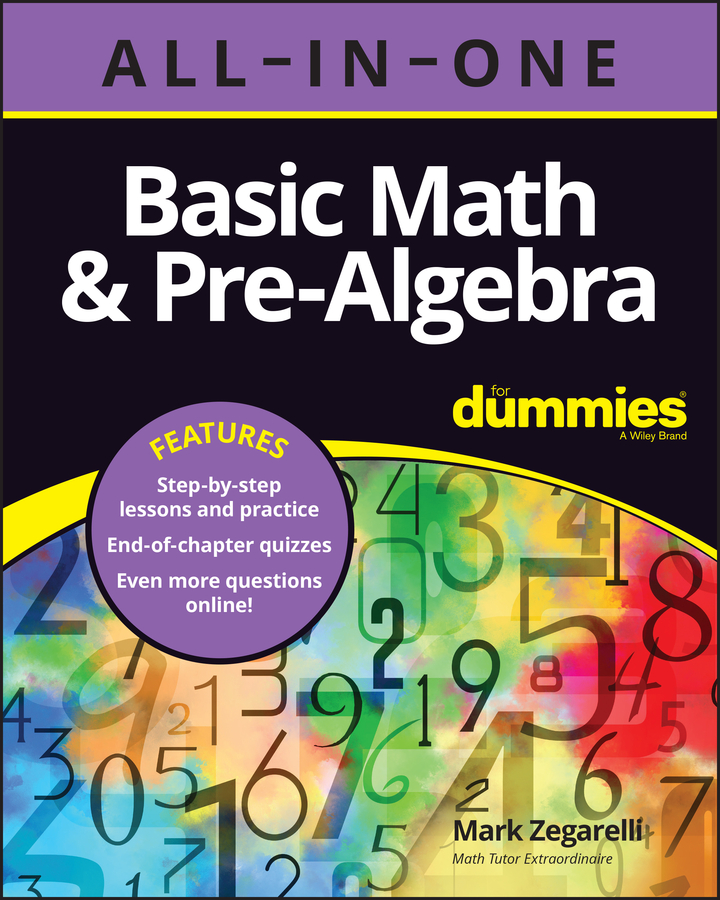Even if fractions look different, they can actually represent the same amount; in other words, one of the fractions will have reduced terms compared to the other. You may need to reduce the terms of fractions to work with them in an equation.

Reducing fractions to their lowest terms involves division. But because you can’t always divide, reducing takes some finesse.

Here you will learn the formal way to reduce fractions, which works in all cases. Then you will learn a more informal way that you can use after you’re more comfortable.

## Method 1: Reduce fractions the formal way

Reducing fractions the formal way relies on an understanding of how to break down a number into its prime factors.

Here’s how to reduce a fraction:

1. Break down both the numerator (top number) and denominator (bottom number) into their prime factors.

For example, suppose you want to reduce the fraction 12/30. Break down both 12 and 30 into their prime factors: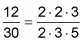2. Cross out any common factors.

In this example, you cross out a 2 and a 3, because they’re common factors — that is, they appear in both the numerator and denominator: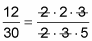3. Multiply the remaining numbers to get the reduced numerator and denominator.

This shows you that the fraction 12/30 reduces to 2/5: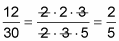As another example, here’s how you reduce the fraction 32/100: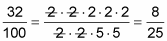This time, cross out two 2s from both the top and the bottom as common factors. The remaining 2s on top, and the 5s on the bottom, aren’t common factors. So the fraction 32/100 reduces to 8/25.

## Method 2: Reduce fractions the informal way

Here’s an easier way to reduce fractions after you get comfortable with the concept:
1. If the numerator (top number) and denominator (bottom number) are both divisible by 2 — that is, if they’re both even — divide both by 2.

For example, suppose you want to reduce the fraction 24/60. The numerator and the denominator are both even, so divide them both by 2: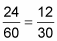2. Repeat Step 1 until the numerator or denominator (or both) is no longer divisible by 2.

In the resulting fraction, both numbers are still even, so repeat the first step again: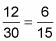3. Repeat Step 1 using the number 3, and then 5, and then 7, continuing testing prime numbers until you’re sure that the numerator and denominator have no common factors.

Now, the numerator and the denominator are both divisible by 3, so divide both by 3: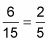Neither the numerator nor the denominator is divisible by 3, so this step is complete. At this point, you can move on to test for divisibility by 5, 7, and so on, but you really don’t need to. The numerator is 2, and it obviously isn’t divisible by any larger number, so you know that the fraction 24/60 reduces to 2/5.